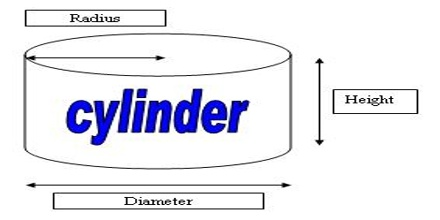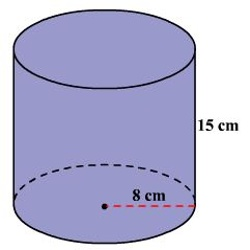A cylinder is a simple geometric shape with two equally-sized and parallel circular bases. Volume of a Cylinder means the number of cubic units that will exactly fill a cylinder

The volume of a cylinder equals the (area of the base)*height = π r2 h

The volume of a 3 -dimensional solid is the amount of space it occupies. Volume is measured in cubic units (in3, ft3,c m3, m3 etc cetera).How to find the volume of a cylinder

Although a cylinder is technically not a prism, it shares many of the properties of a prism. Like prisms, the volume is found by multiplying the area of one end of the cylinder (base) by its height.

Since the end (base) of a cylinder is a circle, the area of that circle is given by the formula:

area = π r2

Multiplying by the height h we get

volume = π r2 h

where:

• π is Pi, approximately 3.142
• r is the radius of the circular end of the cylinder
• h height of the cylinder.

Example:

Find the volume of the cylinder shown. Round to the neatest cubic centimeter.Solution

The formula for the volume of a cylinder is, volume, V = πr2h .

The radius of the cylinder is 8 cm and the height is 15 cm.

Substitute 8 for r and 15 for h in the formula V = πr2h

so, V = 3.1416 x 82 x 15

≈ 3016

Therefore, the volume of the cylinder is about 3016 cubic centimeters.